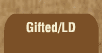Mathematics and Dyslexia

Tips for Learning the Times Tables

Tools for the Times Tables & Workbook

Home > Math > times tables practice > Test: 9, 4, 5, 2, 10, 1 and 0 tables

1. 9 x 9=
2. 5x 5=
3. 4 x 4=
4. 2 x 2=
5. 1 x 1=
6. 7 x 5=
7. 9 x 8=
8. 6 x 2=
9. 10 x 5=
10. 0 x 9=
11. 3 x 5=
12. 9 x 6=
13. 1 x 7=
14. 2 x 8=
15. 7 x 0=
16. 5 x 4=
17. 4 x 6=
18. 9 x 4=
19. 3 x 10=
20. 2 x 1=
21. 6 x 5=
22. 1 x 4=
23. 10 x 2=
24. 8 x 1=
25. 2 x 5=
26. 9 x 5=
27. 2 x 3=
28. 3 x 9=
29. 4 x 0=
30. 7 x 4=
31. 10 x 9=
32. 7 x 2=
33. 5 x 1=
34. 8 x 4=
35. 6 x 0=
36. 10 x 8=
37. 10 x 10=
38. 1 x 9=
39. 0 x 10
40. 9 x 7=
41. 10 x 4=
42. 9 x 3=
43. 5 x 4=
44. 7 x 10=
45. 1 x 6=
46. 10 x 5=
47. 0 x 8=
48. 3 x 1=
49. 4 x 3=
50. 4 x 9=